What is a three terminal capacitor and what are the advantages?

Capacitors are divided into electrolytic capacitors, ceramic capacitors, tantalum capacitors and so on. Ceramic capacitors are widely used in mobile smart products, which are divided into three-terminal capacitors and two-terminal capacitors.

People often say that three-terminal capacitors have good high-frequency characteristics. As a hardware engineer, do you know about three-terminal capacitors?

The following figure is a physical comparison diagram of a two-terminal capacitor and a three-terminal capacitor: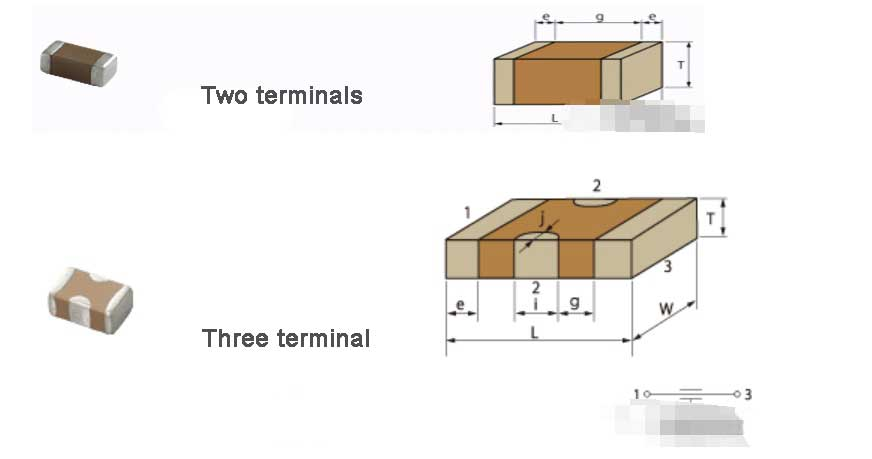The ideal capacitor, as the frequency increases, the impedance becomes lower and lower. See the impedance frequency curve in the figure below.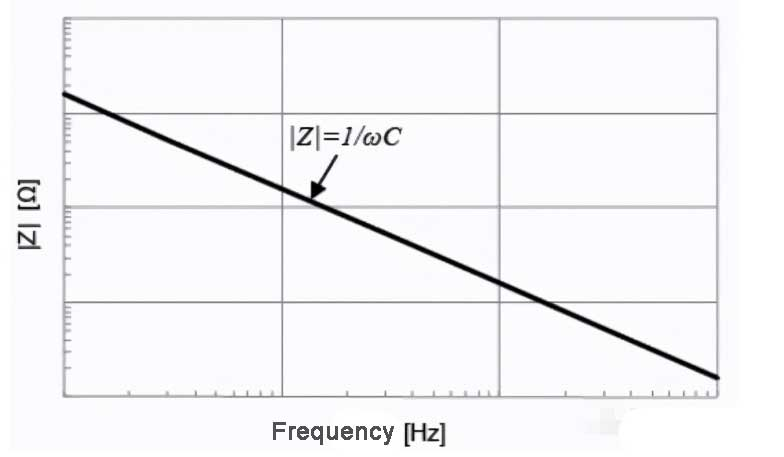However, the actual capacitor has parasitic parameters. The following figure is a simplified equivalent model of the capacitor. Due to the existence of the series equivalent resistance ESR and the series equivalent inductance ESL, the impedance frequency characteristics of the capacitor have undergone tremendous changes.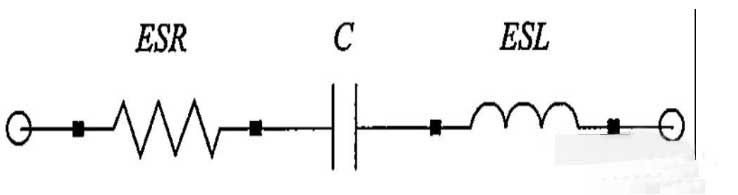The following figure shows the impedance frequency characteristics of the actual capacitor. We can see that in the low frequency band, the capacitor plays a leading role, and the impedance decreases as the frequency increases. However, in the high frequency band, the inductance plays a leading role, and the impedance increases with the increase in frequency. Exactly what we don’t want to see.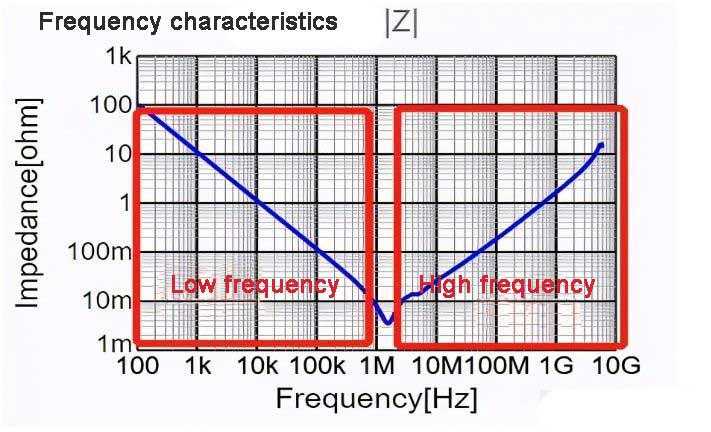The so-called three-terminal capacitor has good high-frequency characteristics, that is, its low ESL.

Let’s compare the impedance difference between the 22uf two-terminal capacitor and the three-terminal capacitor. It can be seen that the impedance of the two-terminal capacitor is about 3mΩ at 1.05Mhz, the resonance frequency of the three-terminal capacitor is higher, and the impedance is only about 2mΩ at 3Mhz; the most important high-frequency part, the two-terminal capacitance even exceeds 1Ω at 1Ghz, and the three-terminal capacitor The terminal capacitance is only 110mΩ.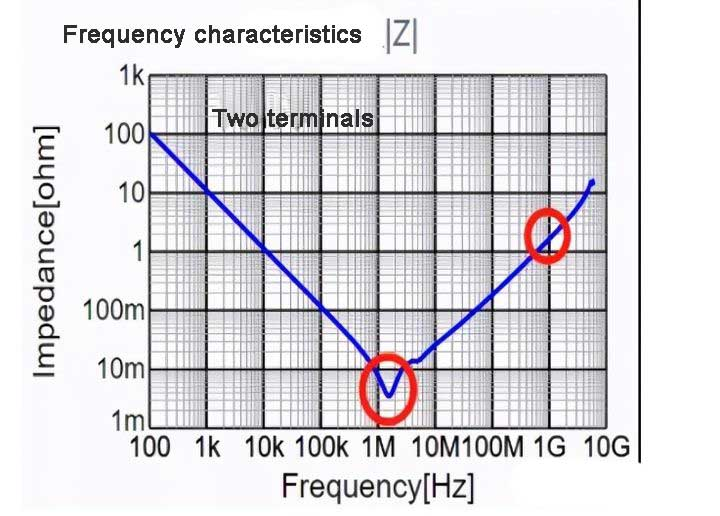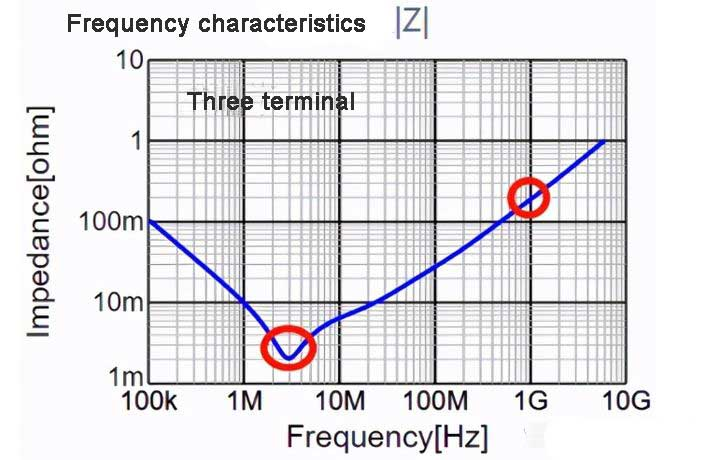So why are the high frequency characteristics of three-terminal capacitors good?

The same question: Why is the ESL of a three-terminal capacitor small?

That is because the three-terminal capacitor has a special structure, which shortens the current path and makes the ESL have parallel characteristics, which in turn reduces the ESL and makes the high-frequency characteristics good.